# Power vs. Torque

In this article we are going to understand how the engine torque is produced, how engine power is calculated and what is a torque and power curve. Also, we are going to have a look at the engine torque and power maps (surfaces).

By the end of the article, the reader will be able to understand the difference between torque and power, how they affect the longitudinal dynamics of the vehicle and how to interpret torque and power curves at full load.

### Definition of torque

Torque can be regarded as a turning force applied on an object. Torque (vector) is the cross product between a force (vector) and a distance (scalar). The distance, also called the lever arm, is measured between the force and the turning point. Similar to a force, torque is a vector and is defined by an amplitude and a direction of rotation.

Imagine that you want to tighten/loosen the bolts of a wheel. Pushing or pulling the handle of the wrench connected to a nut or bolt, produces a torque (turning force) that loosens or tightens the nut or bolt.

The torque T [Nm] is the product of the force F [N] and the length of the lever arm a [m].

$\bbox[#FFFF9D]{T = F \cdot a}$

In order to increase the magnitude of the torque we can either increase the force, the length of the lever arm or both.

Example: Calculate the torque obtained on the bolt if the arm of the wrench has 0.25 m and the applied force is 100 N (which is approx. equivalent with a pushing force of 10 kg)

$T = 100 \cdot 0.25 = 25 \text{ Nm}$

The same torque could be obtained if the lever arm was 1 m and the force only 25 N.

The same principle applies to internal combustion engines. The torque at the crankshaft is produced by the force applied on the conrod journal through the connecting rod.

The torque T will be produce at the crankshaft on each conrod journal, every time the piston is in the power stroke. The lever arm a in this case is the crank radius (offset).

The magnitude of the force F depends on the combustion pressure within the cylinder. The higher the pressure in the cylinder, the higher the force on the crankshaft, the higher the output torque.

The length of the lever arm has impact on the overall engine balance. Increasing it too much can lead to engine imbalance, which results in higher forces in the crankshaft journals.

Example: Calculate the torque at the crankshaft for an engine with the following parameters:

 Cylinder bore, B [mm] 85 Cylinder pressure, p [bar] 12 Crank offset, a [mm] 62

First, we calculate the area of the piston (assuming the the piston head is flat and its diameter is equal with the bore of the cylinder):

$A_p = \frac{\pi B^2}{4}=\frac{\pi \cdot 0.085^2}{4}=0.0056745 \text{ m}^2$

Second, we’ll calculate the force applied to the piston. To get the force in N (Newton), we’ll use the pressure converted in Pa (Pascal).

$F = p \cdot A_p = 120000 \cdot 0.0056745 = 680.94021 \text{ N}$

Assuming that all the force in the piston goes into the connecting rod, the torque is calculated as:

$T = F \cdot a = 680.94021 \cdot 0.062 = 42.218293 \text{ Nm}$

The standard unit of measurement for torque is N·m (Newton meter). Especially in the USA, the unit of measurement for engine torque is lbf·ft (foot-pounds). The conversion between N·m and lbf·ft is:

$\begin{split} 1 \text{ lbf} \cdot \text{ft} &= 1.355818 \text{ N} \cdot \text{m}\\ 1 \text{ N} \cdot \text{m} &= 0.7375621 \text{ lbf} \cdot \text{ft} \end{split}$

For our particular example, the torque in imperial units (USA) is:

$T = 42.218293 \cdot 0.7375621 = 31.138615 \text{ lbf} \cdot \text{ft}$

Torque T [N] can also be expressed as a function of the mean effective pressure of the engine.

$T = \frac{p_{me} V_d}{2 \pi n_r}$

where:
pme [Pa] – mean effective pressure
Vd [m3] – engine displacement (volume)
nr [-] – number of crankshaft rotations for a complete engine cycle (for a 4-stroke engine nr = 2)

### Definition of power

In physics, power is the work done in time or, with other words, is the rate of doing work. In rotational systems, power P [W] is the product of the torque T [Nm] and angular velocity ω [rad/s].

$\bbox[#FFFF9D]{P = T \cdot \omega}$

The standard unit of measurement for power is W (Watt) and for rotational speed is rad/s (radian per second). Most of the vehicle manufacturers are providing the power of the engine in bhp (brake horse power) and the rotational speed in rpm (rotations per minute). Therefore, we are going to use conversion formulas for both rotational speed and power.

To convert from rpm to rad/s, we use:

$\omega \text{ [rad/s]} = N \text{ [rpm]} \cdot \frac{\pi}{30}$

To convert from rad/s to rpm, we use:

$N \text{ [rpm]} = \omega \text{ [rad/s]} \cdot \frac{30}{\pi}$

The engine power can also be measured in kW instead of W for a more compact value. To convert from kW to bhp and reverse, we use:

$\begin{split} P \text{ [bhp]} &= 1.36 \cdot P \text{ [kW]}\\ P \text{ [kW]} &= \frac{P \text{ [bhp]}}{1.36} \end{split}$

In some cases you might find HP (Horse Power) instead of bhp as unit of measurement for power.

Having rotational speed measured in rpm and torque in Nm, the formula to calculate power is:

$\begin{split} P \text{ [kW]} &= \frac{\pi \cdot N \text{ [rpm]} \cdot T \text{ [Nm]}}{30 \cdot 1000}\\ P \text{ [HP]} &= \frac{1.36 \cdot \pi \cdot N \text{ [rpm]} \cdot T \text{ [Nm]}}{30 \cdot 1000} \end{split}$

Example. Calculate the engine power in both kW and HP, if the engine torque is 150 Nm and engine speed is 2800 rpm.

$\begin{split} P &= \frac{\pi \cdot 2800 \cdot 150}{30 \cdot 1000} = 44 \text{ kW}\\ P &= \frac{1.36 \cdot \pi \cdot 2800 \cdot 150}{30 \cdot 1000} = 59.8 \text{ HP} \end{split}$

### Engine dynamometer

Engine speed is measured using a sensor on the crankshaft (flywheel). Ideally, to calculate power, we should also measure the torque at the crankshaft with a sensor. Technically, this is possible but not applied in the automotive industry. Because of the operating conditions of the crankshaft (temperatures, vibrations), measuring engine torque with a sensor is not a reliable technique. Also, the cost of a torque sensor is quite high. Therefore, engine torque is measured on the full range of speed and load, using a dynamometer (test bench), and mapped (stored) into the engine control unit.

The dynamometer is basically a brake (mechanical, hydraulic or electrical) which absorbs the power produced by the engine. The most used and best type of dynamometer is the electric dynamometer. This is actually an electric machine that can be operated as a generator or motor. By varying the generator’s load torque, the engine can be put in any operating point (speed and torque). Also, with the engine at fuel cut (no fuel injection), the generator can be run as an electric motor to spin the engine. This way engine friction and pumping torque losses can be measured.

For an electric dynamometer, the rotor is connected to the crankshaft. The link between rotor and stator is electromagnetic. The stator is fixed through a lever arm to a load cell. To balance the rotor, the stator will push against the load cell. The torque T is calculated by multiplying the force F measured in the load cell with the length of the lever arm a.

$T = F \cdot a$

The engine parameters: brake torque, brake horse power (bhp) or brake specific fuel consumption (BSFC) contain the keyword “brake” because a dynamometer (brake) is used to measure them.

What comes out from a dynamometer engine test are torque maps (surfaces) which give the value of the engine torque at a specific engine speed and load (stationary operating points). The load of the engine is equivalent to the position of the accelerator pedal.

Example of torque map for a gasoline, spark ignition (SI) engine:

 Engine torque [Nm] Accelerator pedal position [%] 5 10 20 30 40 50 60 100 Engine speed [rpm] 800 45 90 107 109 110 111 114 116 1300 60 105 132 133 134 136 138 141 1800 35 89 133 141 142 144 145 149 2300 19 70 133 147 148 150 151 155 2800 3 55 133 153 159 161 163 165 3300 0 41 126 152 161 165 167 171 3800 0 33 116 150 160 167 170 175 4300 0 26 110 155 169 176 180 184 4800 0 18 106 155 174 179 185 190 5300 0 12 96 147 167 175 181 187 5800 0 4 84 136 161 170 175 183 6300 0 0 72 120 145 153 159 171

Example of power map for a gasoline, spark ignition (SI) engine:

 Engine power [HP] Accelerator pedal position [%] 5 10 20 30 40 50 60 100 Engine speed [rpm] 800 5 10 12 12 13 13 13 13 1300 11 19 24 25 25 25 26 26 1800 9 23 34 36 36 37 37 38 2300 6 23 44 48 48 49 49 51 2800 1 22 53 61 63 64 65 66 3300 0 19 59 71 76 78 78 80 3800 0 18 63 81 87 90 92 95 4300 0 16 67 95 103 108 110 113 4800 0 12 72 106 119 122 126 130 5300 0 9 72 111 126 132 137 141 5800 0 3 69 112 133 140 145 151 6300 0 0 65 108 130 137 143 153

The electronic control module (ECM) of an ICE has the torque map stored in the memory. It calculates (interpolates) the engine torque function of the current engine speed and load. In the ECM, the load is expressed as intake manifold pressure for gasoline (spark ignition, SI) engines and injection time or fuel mass for diesel (compression ignition, CI) engines. The engine torque calculation strategy has corrections based on temperature and intake air pressure.

Plotting the torque and power data, function of engine speed and load, gives the following surfaces:

For a better interpretation of the torque and power maps, a 2-D torque line can be plotted for a fixed value of the accelerator pedal position.

### Engine torque and power at full load

As you have seen, the torque and power of an internal combustion engine depend on both engine speed and load. Usually, engine manufacturers are publishing the torque and curve characteristics (curves) at full load (100% accelerator pedal position). Full load torque and power curves highlight the maximum torque and power distribution through the whole range of engine speed.

The shape of the above torque and power curves are not from a real engine, the scope being to explain the main parameters. Nevertheless, the shapes are similar to the real characteristics of a spark ignited (gasoline), port injection, atmospheric engine.

Engine speed Ne [rpm] is characterized by four main points:

Nmin – is the minimum stable engine speed at full load
NTmax – is the engine speed at maximum engine torque
NPmax – is the engine speed at maximum engine power; also called rated engine speed
Nmax – is the maximum stable engine speed

At minimum speed, the engine should run smoothly, without oscillations or stalling. The engine should also allow operation at the maximum speed without any structural damage.

The full load engine torque curve Te [Nm] is characterized by four points:

T0 – engine torque at minimum engine speed
Tmax – maximum engine torque (peak torque or rated torque)
TP – engine torque at maximum engine power
TM – engine torque at maximum engine speed

Depending on the type of intake air (atmospheric or turbocharged) the peak torque can be either a point or a line. For turbocharged or supercharged engines, maximum torque can be kept constant between two engine speed values.

The full load engine power curve Pe [HP] is characterized by four points:

P0 – engine power at minimum engine speed
Pmax – maximum engine power (peak power or rated power)
PT – engine power at maximum engine torque
PM – engine power at maximum engine speed

The area between minimum engine speed Nmin and maximum torque engine speed NTmax is called low end torque zone. The higher the torque in this area, the better the launch/acceleration capabilities of the vehicle. When the engine is operating in this area, at full load, if the road resistance increase, the engine speed will decrease, which will result in a drop of engine torque and an engine stall. For this reason, this area is also called unstable torque region.

The area between maximum torque engine speed NTmax and maximum power engine speed NPmax is called the power band. During vehicle acceleration, for best performance, the gearshift (up) should be performed at maximum engine power. Depending on the gear ratios of the gearbox, after the gearshift, the selected gear will drop the engine speed at maximum torque, which will give optimum acceleration. Shifting the gears at maximum engine power will keep the engine speed within the power band.

The area between maximum power engine speed NPmax and maximum engine speed Nmax is called high end torque zone. Higher torque results in higher output power, which translates in higher maximum vehicle speed and better acceleration at high speed.

When the engine speed is kept between maximum torque engine speed NTmax and maximum engine speed Nmax, if the vehicle road resistance increases, the engine speed will drop and the output torque will increase, thus compensating for the road load increase. For this reason, this area is called the stable torque region.

Below you can find some full load torque and power curves examples for different types of engines. Notice the shape of the curves function of the type of the engine (spark ignited or compression ignited) and type of air intake (atmospheric or turbo(super)charged).

### Honda 2.0 engine torque and power at full load

 Cylinders architecture 4 in-line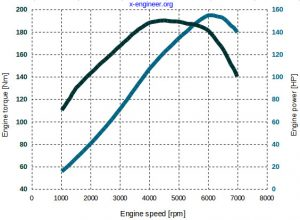Image: Honda 2.0 SI engine – torque and power curves at full load Fuel gasoline (SI) Engine capacity [cm3] 1998 Fuel injection valve port Air intake atmospheric Valve timing variable Tmax [Nm] 190 NTmax [rpm] 4500 Pmax [HP] 155 NPmax [rpm] 6000 Nmax [rpm] 6800

### Saab 2.0T engine torque and power at full load

 Cylinders architecture 4 in-line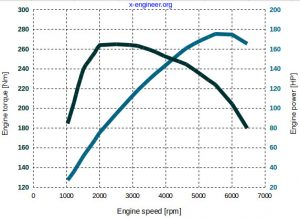Image: Saab 2.0T SI engine – torque and power curves at full load Fuel gasoline (SI) Engine capacity [cm3] 1998 Fuel injection valve port Air intake turbocharged Valve timing fixed Tmax [Nm] 265 NTmax [rpm] 2500 Pmax [HP] 175 NPmax [rpm] 5500 Nmax [rpm] 6300

### Audi 2.0 TFSI engine torque and power at full load

 Cylinders architecture 4 in-line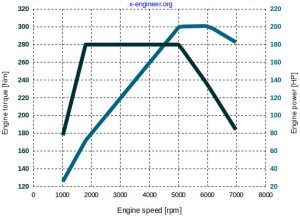Image: Audi 2.0 TFSI SI engine – torque and power curves at full load Fuel gasoline (SI) Engine capacity [cm3] 1994 Fuel injection direct Air intake turbocharged Valve timing fixed Tmax [Nm] 280 NTmax [rpm] 1800 – 5000 Pmax [HP] 200 NPmax [rpm] 5100 – 6000 Nmax [rpm] 6500

### Toyota 2.0 D-4D engine torque and power at full load

 Cylinders architecture 4 in-line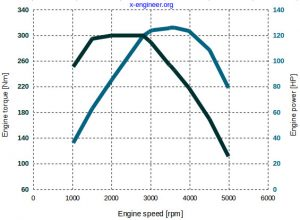Image: Toyota 2.0 CI engine – torque and power curves at full load Fuel diesel (CI) Engine capacity [cm3] 1998 Fuel injection direct Air intake turbocharged Valve timing fixed Tmax [Nm] 300 NTmax [rpm] 2000 – 2800 Pmax [HP] 126 NPmax [rpm] 3600 Nmax [rpm] 5200

### Mercedes-Benz 1.8 Kompressor engine torque and power at full load

 Cylinders architecture 4 in-line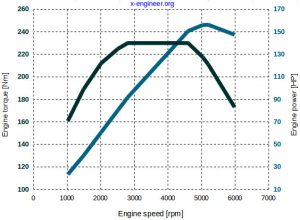Image: Mercedes Benz 1.8 Kompressor SI engine – torque and power curves at full load Fuel gasoline Engine capacity [cm3] 1796 Fuel injection valve port Air intake supercharged Valve timing fixed Tmax [Nm] 230 NTmax [rpm] 2800 – 4600 Pmax [HP] 156 NPmax [rpm] 5200 Nmax [rpm] 6250

### BMW 3.0 TwinTurbo engine torque and power at full load

 Cylinders architecture 6 in-line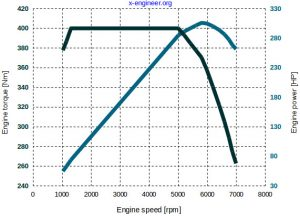Image: BMW 3.0 TwinTurbo SI engine – torque and power curves at full load Fuel gasoline Engine capacity [cm3] 2979 Fuel injection direct Air intake dual-stage turbocharged Valve timing variable Tmax [Nm] 400 NTmax [rpm] 1300 – 5000 Pmax [HP] 306 NPmax [rpm] 5800 Nmax [rpm] 7000

### Mazda 2.6 rotary engine torque and power at full load

 Cylinders architecture 2 Wankel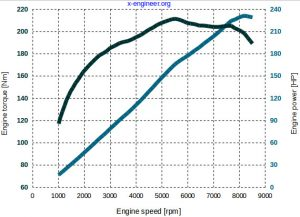Image: Mazda 2.6 SI engine – torque and power curves at full load Fuel gasoline Engine capacity [cm3] 1308 (2616) Fuel injection valve port Air intake atmospheric Valve timing fixed Tmax [Nm] 211 NTmax [rpm] 5500 Pmax [HP] 231 NPmax [rpm] 8200 Nmax [rpm] 9500

### Porsche 3.6 engine torque and power at full load

 Cylinders architecture 6 flat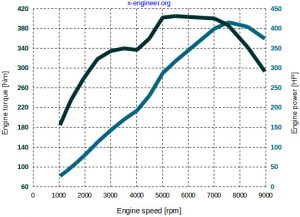Image: Porsche 3.6 SI engine – torque and power curves at full load Fuel gasoline Engine capacity [cm3] 3600 Fuel injection valve port Air intake atmospheric Valve timing variable Tmax [Nm] 405 NTmax [rpm] 5500 Pmax [HP] 415 NPmax [rpm] 7600 Nmax [rpm] 8400

Key statements to keep in mind regarding engine power and torque:

Torque

• torque is a component of power
• torque can be increased by increasing the mean effective pressure of the engine or by lowering the torque losses (friction, pumping)
• having a lower maximum torque distributed on a range of engine speeds its better from the traction point of view than having a higher maximum torque point
• low end torque is very important for the launch capabilities of the vehicles
• high torque is beneficial in off-road situation, when the vehicle is operated at high road gradients but low speed

Power

• engine power depends on both torque and speed
• power can be increased by increasing the torque or the engine speed
• high power is important for high vehicle speeds, the higher the maximum power the higher the maximum speed of the vehicle
• engine power distribution at full load, through the engine speed range, affects the acceleration capability of the vehicle at high speeds
• for best acceleration performance, a vehicle should be operated in the power band, between maximum engine torque and power

For any questions or observations regarding this tutorial please use the comment form below.

Don’t forget to Like, Share and Subscribe!

#### Ad Blocker Detected

Dear user, Our website provides free and high quality content by displaying ads to our visitors. Please support us by disabling your Ad blocker for our site. Thank you!